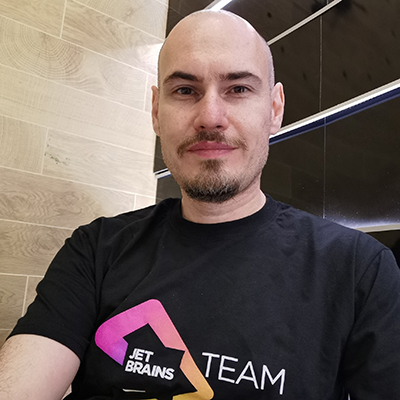RU / Day 2 / 18:00 / Track 1

A wide variety of tools can be used to study theoretical computer science. Perhaps, most of the time the induction theorems are to be proved, yet there is always a place for programming.

In this talk, we'll discuss one approach to the study of theoretical informatics (and more specifically, the theory of algorithms), in which programming in JavaScript becomes the main activity of the student. We will walk through the main sections of the theory and see how Javascript can help us.

To start with, we'll introduce three calculation models (λ calculation, recursive functions and Turing machines), spin them a little to understand what is what, and then try to apply to the theory of computability (where the existence of insoluble problems is proved) and the theory of complexity of calculations (where the problems are classified by the resources level required to solve them — time and memory). Not that Javascript allows you to do basic work in theoretical computer science, but it is able to illustrate important concepts and techniques, and that's exactly what we'll use it for. There will also be a lot of programming exercises. In Javascript! Join us in learning theoretical computer science with our favorite programming language!